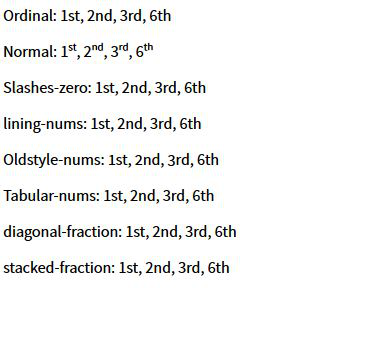Related Articles

# CSS font-variant-numeric Property

• Last Updated : 16 Jul, 2020

The font-variant-numeric property of CSS is used to control the usage of alternate glyphs. This is done in terms of units or markers such as numbers or fractions.

Syntax:

Attention reader! Don’t stop learning now. Get hold of all the important HTML concepts with the Web Design for Beginners | HTML  course.

`font-variant-numeric: value`

Property values:

• normal: Using normal will remove each effect of font-variant-numeric property.
• ordinal: This value directly indicates the open type values i.e ordn. The term makes use of special glyphs for ordinal markers.
• slashed-zero: Slashed-zero used a zero with a slash. This property values prove to be very useful while distinguishing between 0 and O.
• lining-nums: Lining nums property corresponds to the open type values i.e lnum. This keyword activates the numbers lying on the baseline.
• oldstyle-nums: Oldstyle-nums property corresponds to the open type values i.e onum. This keyword activates the set of Figures where some numbers have descendent.
• proportional-nums: This property activates those norms where not every number is of same size. Its Open type value is pnum.
• tabular-nums: Tabular-nums open type value is tnum. It activates those set of figures where set of numbers is of the same size.
• diagonal-fractions: It open type value is frac. This activates those set of figures where numerator and denominator are made smaller and are separated by slash.
• stacked-fractions: It open type value is arac. This activates those set of figures where numerator and denominator are made smaller, stacked, and are separated by horizontal line.

Example:

 ```<``html` `lang``=``"en"``>``    ``<``head``>``        ``<``meta` `charset``=``"UTF-8"` `/>``        ``<``meta` `name``=``"viewport"` `              ``content="``width``=``device``-width,``                       ``initial-scale``=``1``.0" />``        ``<``link``            ``href=``"https://fonts.googleapis.com/css2?``family``=``Source``+Sans+Pro:ital,``              ``wght@0, 200;0, 400;1, 400&``display``=``swap``"``            ``rel``=``"stylesheet"``        ``/>``        ``<``title``>Document``    ````    ``<``style``>``        ``* {``            ``font-family: "Source Sans Pro";``        ``}``        ``.value1 {``            ``font-variant-numeric: normal;``        ``}``        ``.value2 {``            ``font-variant-numeric: ordinal;``        ``}``        ``.value3 {``            ``font-variant-numeric: slashed-zero;``        ``}``        ``.value4 {``            ``font-variant-numeric: lining-nums;``        ``}``        ``.value5 {``            ``font-variant-numeric: oldstyle-nums;``        ``}``        ``.value6 {``            ``font-variant-numeric: tabular-nums;``        ``}``        ``.value7 {``            ``font-variant-numeric: diagonal-fractions;``        ``}``        ``.value7 {``            ``font-variant-numeric: stacked-fractions;``        ``}``    ````    ``<``body``>``        ``<``p``>``            ``<``span``>Ordinal: ``            ``<``span` `class``=``"value1"``>1st, 2nd, 3rd, 6th``        ````        ``<``p``>``            ``<``span``>Normal: ``            ``<``span` `class``=``"value2"``>1st, 2nd, 3rd, 6th``        ````        ``<``p``>``            ``<``span``>Slashes-zero: ``            ``<``span` `class``=``"value3"``>1st, 2nd, 3rd, 6th``        ````        ``<``p` `class``=``"value4"``>``            ``<``span``>lining-nums: ``            ``1st, 2nd, 3rd, 6th``        ````        ``<``p` `class``=``"value5"``>``            ``<``span``>Oldstyle-nums: ``            ``1st, 2nd, 3rd, 6th``        ````        ``<``p` `class``=``"value6"``>``            ``<``span``>Tabular-nums: ``            ``1st, 2nd, 3rd, 6th``        ````        ``<``p` `class``=``"value7"``>``            ``<``span``>diagonal-fraction: ``            ``1st, 2nd, 3rd, 6th``        ````        ``<``p` `class``=``"value7"``>``            ``<``span``>stacked-fraction: ``            ``1st, 2nd, 3rd, 6th``        ````    `````

Output:Supported Browsers: